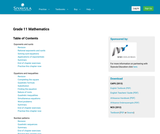Updating search results...

# 1 Result

View
Selected filters:
• SiyavulaUnrestricted Use
CC BY
Rating
3.0 stars

This is a comprehensive math textbook for Grade 11. It can be downloaded, read on-line on a mobile phone, computer or iPad. Every chapter has links to on-line video lessons and explanations. Summary presentations at the end of each chapter offer an overview of the content covered, with key points highlighted for easy revision. Topics covered are: language of mathematics, exponents, surds, error margins, quadratic sequences, finance, quadratic equations, quadratic inequalities, simultaneous equations, mathematical models, quadratic functions and graphs, hyperbolic functions and graphs, exponential functions and graphs, gradient at point, linear programming, geometry, trigonometry, statistics, independent variables, dependent events. This book is based upon the original Free High School Science Text series.

Subject:
Mathematics
Material Type:
Textbook
Provider:
Siyavula
Date Added:
04/12/2012